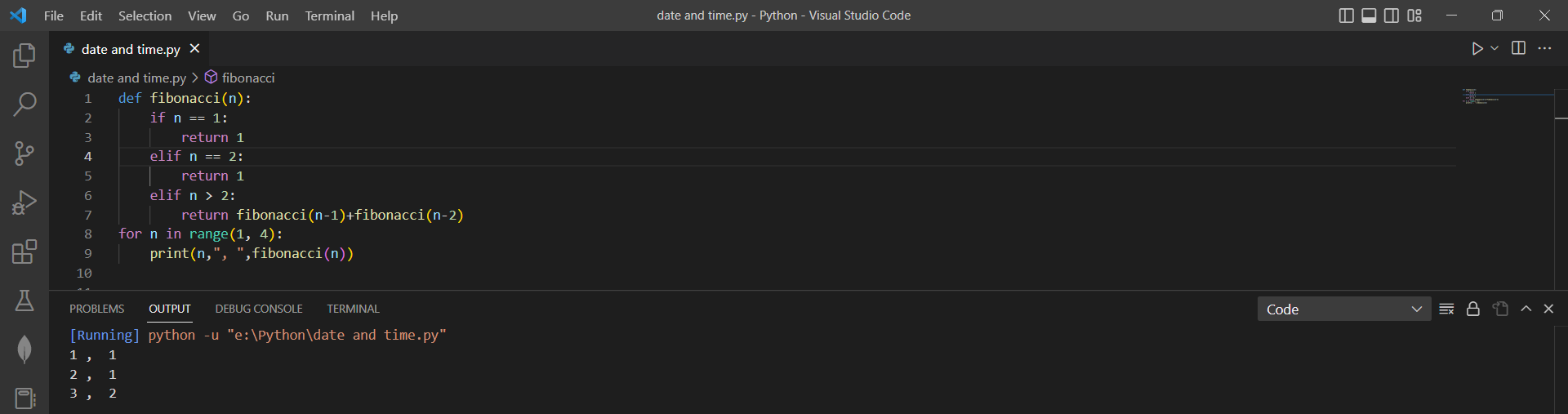# Writing Simple python function using Fibonacci functionby vigneshchennai74Updated: Feb 17, 2023Guide Kit

Fibonacci(n) is a function that takes an integer n as input and calculates the n-th number in the Fibonacci sequence using recursion. The Fibonacci sequence is a number series where every number is the total of the two numbers before that, starting from 0 and 1.

Implementing the fibonacci(n) function first checks if n equals 1 or 2. If so, it returns 1, as the first two numbers in the Fibonacci sequence are both 1. If n is greater than 2, it recursively calls fibonacci(n-1) and fibonacci(n-2) and returns the sum of those two values. A for loop is a type of control flow statement in Python that will allow you to iterate over an element sequence, such as a list, tuple, or string. if and elif are conditional statements in Python that allow you to execute different codes depending on the value of a condition.

Here is an example of how to write a python code to calculate the Fibonacci sequencePreview of the output that you will get on running this code.

### Code

In this code we have used Fibonacci function

1. Copy this code using "Copy" button above and paste it in your Python ide
2. Import the Fibonacci function .
3. Run the code to get the output

I hope you have found this useful. I have added the dependent library and version information in the following section.

I found this code snippet by searching "Writing simple python function " in kandi. you can try any use case.

### Environment Tested

In this solution we have used the following versions. Be mindful to change when working with other versions.

• This solution is created using Python version 3.7.15

Using this solution you'll learn to print the Fibonacci sequence in python with simple Steps. This process also facilities an easy to use, hassle free method to create a hands-on working version of code which would help us to print the Fibonacci sequence in Python.

### Support

1. For any support on kandi solution kits, please use the chat
2. For further learning resources, visit the Open Weaver Community learning page.